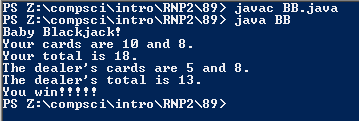# Assignment #89 Baby Blackjack

## Code

```    ///Name: Morgan Kaplan
///Period: 5
///Project Name: Baby Blackjack
///File Name: BB.java

import java.util.Random;

public class BB
{
public static void main( String[] args)
{
Random r=new Random();
int r1=1+r.nextInt(10),r2=1+r.nextInt(10),r3=1+r.nextInt(10),r4=1+r.nextInt(10);

System.out.println( "Baby Blackjack!");

System.out.println( "Your cards are " +r1+ " and " + r2 + ".");
int pt=(r1+r2);

System.out.println( "The dealer's cards are " +r3+ " and " + r4 + ".");
int dt=(r3+r4);
System.out.println("The dealer's total is "+dt+".");

if( pt>dt){
System.out.println("You win!!!!!");}
else{
System.out.println("The dealer wins :(");}
}
}

```

### Picture of Output